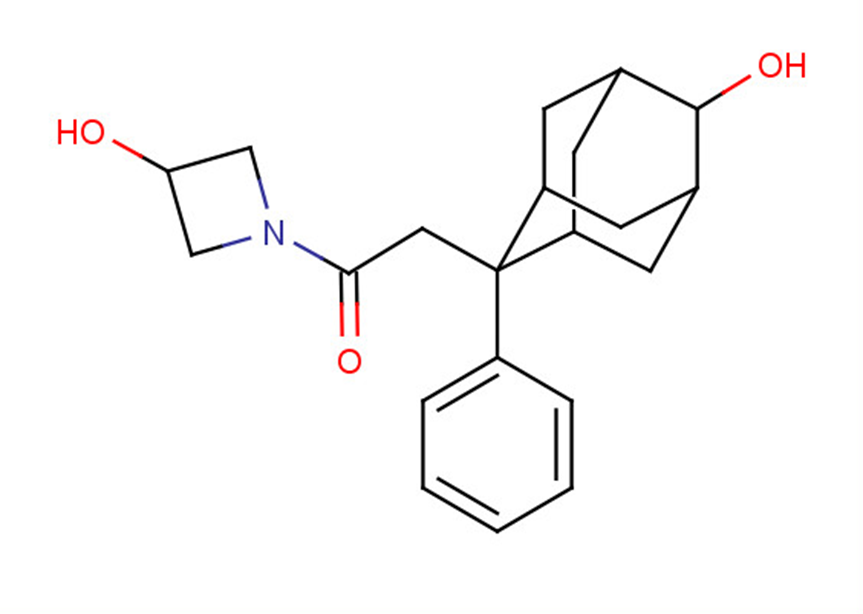# (Rac)-BMS-816336

Catalog No. T12663   CAS T12663

(Rac)-BMS-816336 (Compound 6n) is a racemate of BMS-816336. (Rac)-BMS-816336 is a potent human and mouse 11β-hydroxysteroid <a href="/target/Dehydrogenase" style="display: inline; color: #c13a36">Dehydrogenase</a> type 1 (11β-HSD1) enzyme inhibitor with IC 50 s of 10 nM and 68 nM, respectively. (Rac)-BMS-816336 has good metabolic stability .

All products from TargetMol are for Research Use Only. Not for Human or Veterinary or Therapeutic Use.(Rac)-BMS-816336, CAS T12663
Pack Size Availability Price/USD Quantity
50 mg 12-16 weeks \$ 1,487.00
100 mg 12-16 weeks \$ 2,388.00
Get quote
Purity: 98%
Biological Description
Chemical Properties
Storage & Solubility Information
 Description (Rac)-BMS-816336 (Compound 6n) is a racemate of BMS-816336. (Rac)-BMS-816336 is a potent human and mouse 11β-hydroxysteroid dehydrogenase type 1 (11β-HSD1) enzyme inhibitor with IC 50 s of 10 nM and 68 nM, respectively. (Rac)-BMS-816336 has good metabolic stability . Targets&IC50 mouse 11β-HSD1:68 nM , human 11β-HSD1:10 nM In vivo (Rac)-BMS-816336 (compound 6n) has good metabolic stability with the remaining rates in human, mouse and rats of 88%,82% and 72%, respectively .
 Molecular Weight 341.44 Formula C21H27NO3 CAS No. T12663

#### Storage

Powder: -20°C for 3 years

In solvent: -80°C for 2 years

#### Solubility Information

( < 1 mg/ml refers to the product slightly soluble or insoluble )

## Related Products

Related compounds with same targets

##Dose Conversion

You can also refer to dose conversion for different animals. More

##In vivo Formulation Calculator (Clear solution)

Step One: Enter information below
Dosage
mg/kg
Average weight of animals
g
Dosing volume per animal
ul
Number of animals
Step Two: Enter the in vivo formulation
% DMSO
%
% Tween 80
% ddH2O

##Calculator

Molarity Calculator
Dilution Calculator
Reconstitution Calculation
Molecular Weight Calculator
=
X
X

### Molarity Calculator allows you to calculate the

• Mass of a compound required to prepare a solution of known volume and concentration
• Volume of solution required to dissolve a compound of known mass to a desired concentration
• Concentration of a solution resulting from a known mass of compound in a specific volume
See Example

An example of a molarity calculation using the molarity calculator
What is the mass of compound required to make a 10 mM stock solution in 10 ml of water given that the molecular weight of the compound is 197.13 g/mol?
Enter 197.13 into the Molecular Weight (MW) box
Enter 10 into the Concentration box and select the correct unit (millimolar)
Enter 10 into the Volume box and select the correct unit (milliliter)
Press calculate
The answer of 19.713 mg appears in the Mass box

X
=
X

### Calculator the dilution required to prepare a stock solution

Calculate the dilution required to prepare a stock solution
The dilution calculator is a useful tool which allows you to calculate how to dilute a stock solution of known concentration. Enter C1, C2 & V2 to calculate V1.

See Example

An example of a dilution calculation using the Tocris dilution calculator
What volume of a given 10 mM stock solution is required to make 20ml of a 50 μM solution?
Using the equation C1V1 = C2V2, where C1=10 mM, C2=50 μM, V2=20 ml and V1 is the unknown:
Enter 10 into the Concentration (start) box and select the correct unit (millimolar)
Enter 50 into the Concentration (final) box and select the correct unit (micromolar)
Enter 20 into the Volume (final) box and select the correct unit (milliliter)
Press calculate
The answer of 100 microliter (0.1 ml) appears in the Volume (start) box

=
/

### Calculate the volume of solvent required to reconstitute your vial.

The reconstitution calculator allows you to quickly calculate the volume of a reagent to reconstitute your vial.
Simply enter the mass of reagent and the target concentration and the calculator will determine the rest.

g/mol

### Enter the chemical formula of a compound to calculate its molar mass and elemental composition

Tip: Chemical formula is case sensitive: C10H16N2O2 c10h16n2o2

Instructions to calculate molar mass (molecular weight) of a chemical compound:
To calculate molar mass of a chemical compound, please enter its chemical formula and click 'Calculate'.
Definitions of molecular mass, molecular weight, molar mass and molar weight:
Molecular mass (molecular weight) is the mass of one molecule of a substance and is expressed n the unified atomic mass units (u). (1 u is equal to 1/12 the mass of one atom of carbon-12)
Molar mass (molar weight) is the mass of one mole of a substance and is expressed in g/mol.

bottom

## Tech Support

Please see Inhibitor Handling Instructions for more frequently ask questions. Topics include: how to prepare stock solutions, how to store products, and cautions on cell-based assays & animal experiments, etc.